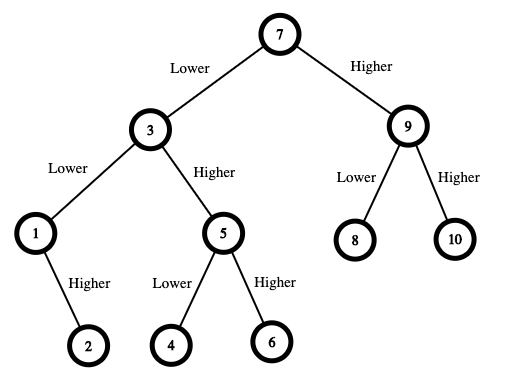### LeetCode• ㊗️
• 大家
• offer
• 多多！

## Problem

We are playing the Guessing Game. The game will work as follows:

• I pick a number between 1 and n.
• You guess a number.
• If you guess the right number, you win the game.
• If you guess the wrong number, then I will tell you whether the number I picked is higher or lower, and you will continue guessing.
• Every time you guess a wrong number x, you will pay x dollars. If you run out of money, you lose the game. Given a particular n, return the minimum amount of money you need to guarantee a win regardless of what number I pick.

Example 1:Input: n = 10
Output: 16
Explanation: The winning strategy is as follows:

- The range is [1,10]. Guess 7. - If this is my number, your total is $0. Otherwise, you pay$7. - If my number is higher, the range is [8,10]. Guess 9. - If this is my number, your total is $7. Otherwise, you pay$9. - If my number is higher, it must be 10. Guess 10. Your total is $7 +$9 = $16. - If my number is lower, it must be 8. Guess 8. Your total is$7 + $9 =$16. - If my number is lower, the range is [1,6]. Guess 3. - If this is my number, your total is $7. Otherwise, you pay$3. - If my number is higher, the range is [4,6]. Guess 5. - If this is my number, your total is $7 +$3 = $10. Otherwise, you pay$5. - If my number is higher, it must be 6. Guess 6. Your total is $7 +$3 + $5 =$15. - If my number is lower, it must be 4. Guess 4. Your total is $7 +$3 + $5 =$15. - If my number is lower, the range is [1,2]. Guess 1. - If this is my number, your total is $7 +$3 = $10. Otherwise, you pay$1. - If my number is higher, it must be 2. Guess 2. Your total is $7 +$3 + $1 =$11.
The worst case in all these scenarios is that you pay $16. Hence, you only need$16 to guarantee a win.


Example 2:

Input: n = 1
Output: 0
Explanation: There is only one possible number, so you can guess 1 and not have to pay anything.


Example 3:

Input: n = 2
Output: 1
Explanation: There are two possible numbers, 1 and 2.
- Guess 1. - If this is my number, your total is $0. Otherwise, you pay$1. - If my number is higher, it must be 2. Guess 2. Your total is $1. The worst case is that you pay$1.


## Code

class Solution {
int[][] dp;
public int getMoneyAmount(int n) {
dp = new int[n + 1][n + 1];
return helper(1, n);
}

private int helper(int i, int j){
if(i >= j) return 0;
if(dp[i][j] != 0) return dp[i][j];

int res = Integer.MAX_VALUE;
for(int x = i; x <= j; x++){
res = Math.min(res, Math.max(helper(i, x - 1), helper(x + 1, j)) + x);
}

dp[i][j] = res;
return res;
}
}